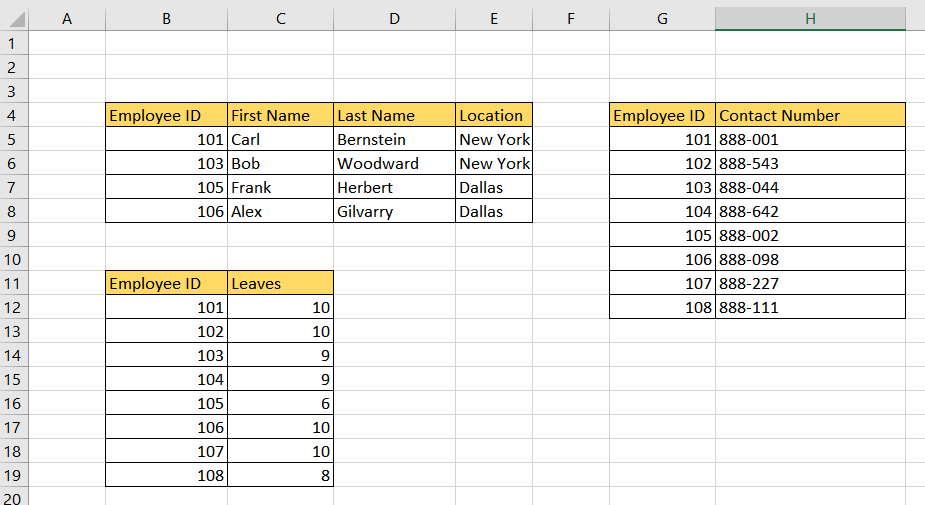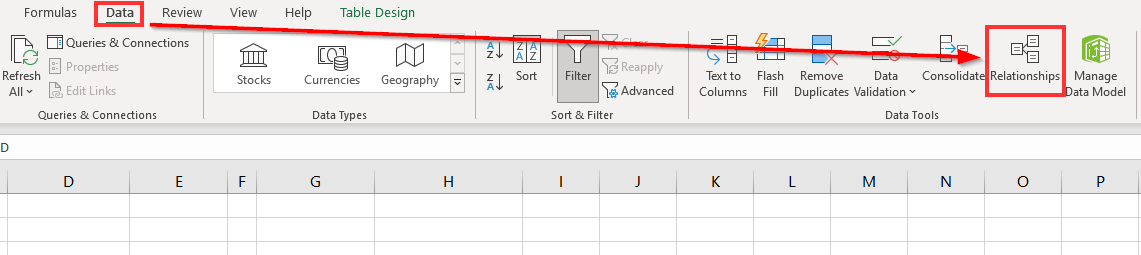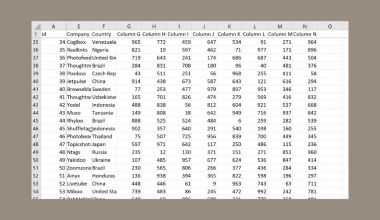# How to Create a Data Model in Excel

This guide will explain all the steps necessary to create a data model in Excel.

Data models are a feature in Excel that allows the user to integrate data coming from multiple sources.

You may already be using a data model without realizing it. Data models are created implicitly when the user imports two or more tables simultaneously.

You can create a model explicitly using Excel’s Power Pivot add-in to import data.

Data models in Excel are visualized as a collection of tables in a Field List.

Let’s take a look at a quick example of where we can benefit from a data model.

Suppose you have three different tables in your workbook. The first table contains basic employee data such as first name, last name, and location. Each employee also has their corresponding employee ID.

The second table has a list of employee IDs and their corresponding number of leaves. The third table contains a list of employee IDs and the contact number associated with that employee.

We can use the Data Model tool to connect each of these tables together. Since each table has an employee ID field, we can use that value to form a relationship or connection between tables.

Compared to the `LOOKUP` function, the data model features use much less memory. This is essential when working with large amounts of data.

Now that we have a grasp on when to create a data model in Excel, let’s learn how to use it and work on an actual sample spreadsheet.

## A Real Example of Using Data Models in Excel

The following section provides an example of a data model in Excel. We will also go into detail about the formulas and tools used in these examples.

In the example below, we have several tables that we want to connect. While it is possible to add all the data into a single table using `LOOKUP`, `INDEX`, or `VLOOKUP`, there is a much simpler approach.We can use Excel’s Data Model feature to define how each table relates to the other. Connecting our data this way makes it possible to use data from different sources in a PivotTable.Do you want to take a closer look at our examples? You can make your own copy of the spreadsheet above using the link attached below.

If you want to create your own data model, head over to the next section to read how you can do it yourself!

## How to Create a Data Model in Excel

This section will guide you through each step needed to begin adding a data model in Excel. You’ll first learn how to convert a basic dataset into an Excel Table. Afterward, we’ll explain how to define relationships between two or more Excel Tables.

Follow these steps to create a data model in Excel:

1. First, navigate to the Insert tab and click on the Table option.2. Select the range you want to convert into an Excel table. In this guide, we will start by converting the cell range B4:E8 into an Excel table. Check the option labeled ‘My table has headers’ and click on OK.3. The range should now change in appearance. Each header should now have a dropdown arrow that can be used to apply filters and sorting.4. Repeat the previous steps until you have all the tables needed for your data model.5. In the Data tab, click on the Relationships option6. In the Manage Relationships dialog box, click on New to add a new relationship between two tables.7. In the Create Relationship dialog box, select the two tables you want to connect. In this example, we’ll first join the employee_contact and employee_leaves tables.8. Next, indicate the column that will serve as the foreign and primary key for the relationship. In this case, we will have to use the Employee ID field. Click on OK to create the relationship.9. The new relationship should now appear in the Manage Relationships dialog box.10. We can repeat the same process to add more relationships between tables.11. Once you have a data model, you can explore your data using the Power Pivot add-in.These are all the steps needed to create a data model in Excel.

## Frequently Asked Questions (FAQ)

1. Why use a Data Model over a lookup table?
Data models are more effective than a typical lookup table because we can define the relationship between two or more tables. These relationships allow us to use PivotTables using all fields in our Data Model. Data models store the relationships of the data to memory automatically.

This step-by-step guide is a quick introduction to using the Data Model feature in Microsoft Excel.

We’ve explained how to convert a dataset into an Excel table. We can then define a relationship between multiple Excel Tables to form a data model.

Data models are just one example of the many Excel functions you can use in Excel. Our website offers hundreds of other functions and methods to help you get more out of Microsoft Excel.

With so many other Excel functions available, you can find one that is appropriate for your use case.

Subscribe to our newsletter to look into the latest Excel guides and tutorials from us.### Get emails from us about Excel.

Our goal this year is to create lots of rich, bite-sized tutorials for Excel users like you. If you liked this one, you'd love what we are working on! Readers receive ✨ early access ✨ to new content.

##### You May Also Like## How to Populate a List Based on a Cell Value in Excel

This guide will explain how to populate a list based on cell values in Excel. The user can…## How to Create a Win-Loss Sparkline Chart in Excel

This guide will explain how to create a Win-Loss sparkline chart in Excel to visualize positive and negative…## How to Insert a Specified Picture into a UserForm in Excel

This guide will explain how you can insert a specified picture into a UserForm in Excel. UserForms are…## How to Use SetFocus VBA in Excel

The SetFocus method in Excel VBA is useful when you need to program the user’s cursor to focus…